# Compass

What angle are between directions WSW (WestSouthWest) and SSE (SouthSouthEast) on the compass?

Correct result:

x =  90 °

#### Solution:

$x=\frac{360}{16}\mathrm{\mid }11-7\mathrm{\mid }=9{0}^{\circ }$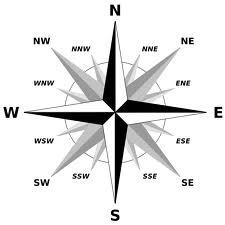We would be pleased if you find an error in the word problem, spelling mistakes, or inaccuracies and send it to us. Thank you!Tips to related online calculators

#### You need to know the following knowledge to solve this word math problem:

We encourage you to watch this tutorial video on this math problem:

## Next similar math problems:

• AnglesThe outer angle of the triangle ABC at the vertex A is 114°12'. The outer angle at the vertex B is 139°18'. What size is the internal angle at the vertex C?
• Special watchFero bought a special watch on the market. They have only one (minute) hand and a display that shows which angle between the hour and minute hand. How many hours it was when his watch showed - the minute hand points to number 2; the display shows 125°?
• Climb in percentageThe height difference between points A and B is 475 m. Calculate the percentage of route climbing if the horizontal distance places A, B is 7.4 km.
• Clock Tower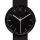What angle is betwenn hands on Clock Tower when show 17 hours and 35 minutes?
• TrianglesFind out whether given sizes of the angles can be interior angles of a triangle: a) 23°10',84°30',72°20' b) 90°,41°33',48°37' c) 14°51',90°,75°49' d) 58°58',59°59',60°3'
• Cu wireCopper wire has a length l = 980 m and diameter d = 8 mm. Calculate the weight if density of copper is ρ = 8500 kg/m3. Result round to one decimal place.
• Two cars 2Two cars started from two positions 87 km distant at the same time in opposite directions at speeds 81 km/h and 75 km/h. What was the distance between them after 2 hours 50 minutes of driving.Between cities A and B is route 13 km long of stúpanie average 7‰. Calculate the height difference of cities A and B.
• Clock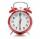What distance will pass end of 8 cm long hour hand for 15 minutes?
• Four prismsQuestion No. 1: The prism has the dimensions a = 2.5 cm, b = 100 mm, c = 12 cm. What is its volume? a) 3000 cm2 b) 300 cm2 c) 3000 cm3 d) 300 cm3 Question No.2: The base of the prism is a rhombus with a side length of 30 cm and a height of 27 cm. The heig
• Steel tubeThe steel tube has an inner diameter of 4 cm and an outer diameter of 4.8 cm. The density of the steel is 7800 kg/m3. Calculate its length if it weighs 15 kg.
• Slope of trackCalculate the average slope (in permille and even in degrees) of the rail tracks between Prievidza (309 m AMSL) and Nitrianske Pravno (354 m AMSL), if the track is 11 km long.
• Map - climb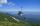On the map of High Tatras in scale 1:11000 are cable car stations in the Tatranska Lomnica and in the Skalnate Pleso with distance 354.6 mm. Altitude of this stations are 949 m and 1760 m. What is average angle of climb of this cable car track?
• Right angled triangle 3Side b = 1.5, hypotenuse angle A = 70 degrees, Angle B = 20 degrees. Find its unknown sides length.
• Propeller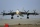The aircraft propeller rotates at an angular speed of 200 rad/s. A) What is the speed at the tip of the propeller if its distance from the axis of rotation is 1.5 m? B) What path does the aircraft travel during one revolution of the propeller at a speed o
• Inclined plane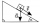The body stays on an inclined plane and exerts a compressive force of 70N on it. Find the angle between the inclined plane and the horizontal if a gravitational force of 100N acts on the body.
• HandsThe clock shows 12 hours. After how many minutes will angle between the hour and minute hand 90°? Consider the continuous movement of both hands hours.